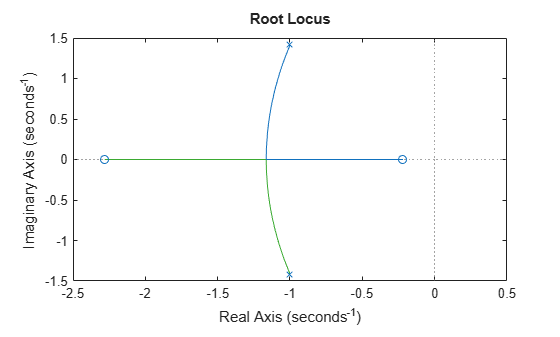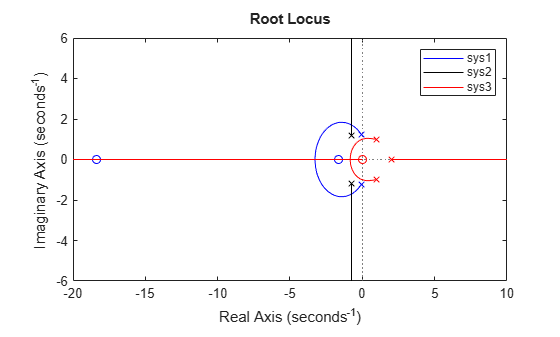# rlocus

Root locus plot of dynamic system

## Syntax

``rlocus(sys)``
``rlocus(sys1,sys2,...)``
``[r,k] = rlocus(sys)``
``r = rlocus(sys,k)``

## Description

example

````rlocus(sys)` calculates and plots the root locus of the SISO model `sys`. The root locus returns the closed-loop pole trajectories as a function of the feedback gain `k` (assuming negative feedback). Root loci are used to study the effects of varying feedback gains on closed-loop pole locations. In turn, these locations provide indirect information on the time and frequency responses.You can use `rlocus` to plot the root locus diagram of any of the following negative feedback loops by setting `sys` as shown below:For instance, if `sys` is a transfer function represented by$sys\left(s\right)=\frac{n\left(s\right)}{d\left(s\right)}$the closed-loop poles are the roots of$d\left(s\right)+kn\left(s\right)=0$The root locus plot depicts the trajectories of closed-loop poles when the feedback-gain `k` varies from 0 to infinity. `rlocus` adaptively selects a set of positive gains `k` to produce a smooth plot. The poles on the root locus plot are denoted by `x` and the zeros are denoted by `o`.```

example

````rlocus(sys1,sys2,...)` plots the root loci of multiple LTI models `sys1, sys2,...` on a single plot. You can specify a color, line style, and marker for each model. For even more plot customization options, see `rlocusplot`.```

example

````[r,k] = rlocus(sys)` returns the vector of feedback gains `k` and the complex root locations `r` for these gains.```

example

````r = rlocus(sys,k)` uses the user-specified vector of feedback gains `k` to output the closed-loop poles `r` that define the root locus plot.```

## Examples

collapse all

For this example. plot the root-locus of the following SISO dynamic system:

`$sys\left(s\right)=\frac{2{s}^{2}+5s+1}{{s}^{2}+2s+3}.$`

```sys = tf([2 5 1],[1 2 3]); rlocus(sys)```The poles of the system are denoted by `x`, while the zeros are denoted by `o` on the root locus plot. You can use the menu within the generated root locus plot to add grid lines, zoom in or out, and also invoke the Property Editor to customize the plot.

For more plot customization options, use `rlocusplot`.

For this example, consider `sisoModels.mat` which contains the following three SISO models:

• `sys1` $-$ a transfer function model

• `sys2` $-$ a state-space model

• `sys3` $-$ a zero-pole-gain model

Load the models from the `mat` file.

`load('sisoModels.mat','sys1','sys2','sys3');`

Create the root locus plot using `rlocus` and specify the color for each system. Also add a legend to the root locus plot.

```rlocus(sys1,'b',sys2,'k',sys3,'r') hold on legend('sys1','sys2','sys3') hold off```The figure contains root locus diagrams for all three systems in the same plot. For more plot customization, see `rlocusplot`.

For this example, consider the following SISO transfer function model:

`$sys\left(s\right)=\frac{3{s}^{2}+1}{9{s}^{3}+7{s}^{2}+5s+6}$`

Use the above transfer function model with `rlocus` to extract the closed-loop poles and associated feedback gain values.

```sys = tf([3 1],[9 7 5 6]); [r,k] = rlocus(sys)```
```r = 3×53 complex -0.9406 + 0.0000i -0.8744 + 0.0000i -0.8685 + 0.0000i -0.8620 + 0.0000i -0.8550 + 0.0000i -0.8475 + 0.0000i -0.8394 + 0.0000i -0.8306 + 0.0000i -0.8212 + 0.0000i -0.8111 + 0.0000i -0.8003 + 0.0000i -0.7888 + 0.0000i -0.7766 + 0.0000i -0.7636 + 0.0000i -0.7500 + 0.0000i -0.7358 + 0.0000i -0.7209 + 0.0000i -0.7055 + 0.0000i -0.6896 + 0.0000i -0.6734 + 0.0000i -0.6569 + 0.0000i -0.6402 + 0.0000i -0.6236 + 0.0000i -0.6071 + 0.0000i -0.5908 + 0.0000i -0.5748 + 0.0000i -0.5593 + 0.0000i -0.5443 + 0.0000i -0.5299 + 0.0000i -0.5161 + 0.0000i -0.5030 + 0.0000i -0.4906 + 0.0000i -0.4789 + 0.0000i -0.4679 + 0.0000i -0.4576 + 0.0000i -0.4480 + 0.0000i -0.4390 + 0.0000i -0.4306 + 0.0000i -0.4229 + 0.0000i -0.4157 + 0.0000i -0.4090 + 0.0000i -0.4029 + 0.0000i -0.3972 + 0.0000i -0.3919 + 0.0000i -0.3871 + 0.0000i -0.3826 + 0.0000i -0.3785 + 0.0000i -0.3748 + 0.0000i -0.3713 + 0.0000i -0.3681 + 0.0000i 0.0814 + 0.8379i 0.0483 + 0.9140i 0.0453 + 0.9212i 0.0421 + 0.9291i 0.0386 + 0.9377i 0.0349 + 0.9470i 0.0308 + 0.9573i 0.0264 + 0.9686i 0.0217 + 0.9809i 0.0167 + 0.9943i 0.0113 + 1.0090i 0.0055 + 1.0251i -0.0006 + 1.0426i -0.0071 + 1.0617i -0.0139 + 1.0826i -0.0210 + 1.1053i -0.0284 + 1.1300i -0.0362 + 1.1568i -0.0441 + 1.1859i -0.0522 + 1.2175i -0.0605 + 1.2515i -0.0688 + 1.2883i -0.0771 + 1.3278i -0.0853 + 1.3703i -0.0935 + 1.4158i -0.1015 + 1.4644i -0.1092 + 1.5162i -0.1167 + 1.5714i -0.1239 + 1.6299i -0.1308 + 1.6920i -0.1374 + 1.7578i -0.1436 + 1.8273i -0.1494 + 1.9006i -0.1549 + 1.9780i -0.1601 + 2.0594i -0.1649 + 2.1452i -0.1694 + 2.2354i -0.1736 + 2.3302i -0.1775 + 2.4299i -0.1810 + 2.5345i -0.1844 + 2.6442i -0.1875 + 2.7594i -0.1903 + 2.8802i -0.1929 + 3.0069i -0.1953 + 3.1397i -0.1976 + 3.2789i -0.1996 + 3.4247i -0.2015 + 3.5775i -0.2032 + 3.7375i -0.2048 + 3.9052i 0.0814 - 0.8379i 0.0483 - 0.9140i 0.0453 - 0.9212i 0.0421 - 0.9291i 0.0386 - 0.9377i 0.0349 - 0.9470i 0.0308 - 0.9573i 0.0264 - 0.9686i 0.0217 - 0.9809i 0.0167 - 0.9943i 0.0113 - 1.0090i 0.0055 - 1.0251i -0.0006 - 1.0426i -0.0071 - 1.0617i -0.0139 - 1.0826i -0.0210 - 1.1053i -0.0284 - 1.1300i -0.0362 - 1.1568i -0.0441 - 1.1859i -0.0522 - 1.2175i -0.0605 - 1.2515i -0.0688 - 1.2883i -0.0771 - 1.3278i -0.0853 - 1.3703i -0.0935 - 1.4158i -0.1015 - 1.4644i -0.1092 - 1.5162i -0.1167 - 1.5714i -0.1239 - 1.6299i -0.1308 - 1.6920i -0.1374 - 1.7578i -0.1436 - 1.8273i -0.1494 - 1.9006i -0.1549 - 1.9780i -0.1601 - 2.0594i -0.1649 - 2.1452i -0.1694 - 2.2354i -0.1736 - 2.3302i -0.1775 - 2.4299i -0.1810 - 2.5345i -0.1844 - 2.6442i -0.1875 - 2.7594i -0.1903 - 2.8802i -0.1929 - 3.0069i -0.1953 - 3.1397i -0.1976 - 3.2789i -0.1996 - 3.4247i -0.2015 - 3.5775i -0.2032 - 3.7375i -0.2048 - 3.9052i ```
```k = 1×53 104 × 0 0.0001 0.0001 0.0001 0.0001 0.0001 0.0001 0.0001 0.0001 0.0001 0.0001 0.0001 0.0002 0.0002 0.0002 0.0002 0.0002 0.0003 0.0003 0.0003 0.0003 0.0004 0.0004 0.0004 0.0005 0.0005 0.0006 0.0006 0.0007 0.0007 0.0008 0.0009 0.0010 0.0011 0.0012 0.0013 0.0014 0.0015 0.0017 0.0018 0.0020 0.0022 0.0024 0.0026 0.0028 0.0031 0.0034 0.0037 0.0041 0.0045 ```

Since `sys` contains 3 poles, the size of the resultant array of poles `r` is 3x53. Each column in `r` corresponds to a gain value from vector `k`. For this example, `rlocus` automatically chose 53 values of `k` from zero to infinity to obtain a smooth trajectory for the three closed-loop poles.

`display(r(:,39))`
``` -0.4229 + 0.0000i -0.1775 + 2.4299i -0.1775 - 2.4299i ```
`display(k(39))`
``` 16.5907 ```

For instance, `r(:,39)` contains the above closed-loop poles for a feedback gain value of 16.5907.

For this example, consider the following SISO transfer function model:

`$sys\left(s\right)=\frac{0.5{s}^{2}-1}{4{s}^{4}+3{s}^{2}+2}$`

Define the transfer function model and required vector of feedback gain values. For this example, consider a set of gain values varying from 1 to 8 with increments of 0.5 and extract the closed-loop pole locations using `rlocus`.

```sys = tf([0.5 -1],[4 0 3 0 2]); k = (1:0.5:5); r = rlocus(sys,k); size(r)```
```ans = 1×2 4 9 ```

Since `sys` contains 4 closed-loop poles, the size of the resultant array of closed-pole locations `r` is 4x9 where the 9 columns correspond to the 9 specific gain values defined in `k`.

You can also visualize the trajectory of the closed-loop poles for the specific gain values in `k` on the root locus plot.

`rlocus(sys,k)`## Input Arguments

collapse all

SISO dynamic system, specified as one of the following:

Feedback gain values that pertain to pole locations, specified as a vector. The feedback gains define the trajectory of the poles thereby affecting the shape of the root locus plot.

## Output Arguments

collapse all

Closed-loop pole locations of `sys` corresponding to each value in `k`, returned as an `n`-by-`m` array, where `n` is the number of closed-loop poles of `sys` and `m = max(length(k))`.

Feedback gain values that pertain to pole locations, returned as a vector. The feedback gains define the trajectory of the poles thereby affecting the shape of the root locus plot. When `k` is not defined by the user, `rlocus` adaptively selects a set of positive gains `k` between zero and infinity, to produce a smooth plot.